cancel
Showing results for
Did you mean:## Calculating subtotals for the summary table using ROLLUPGROUP & SUMMARIZE

Let's build a product summary table for our initial table with orders.

Table Orders:Table Products:Relationships between tables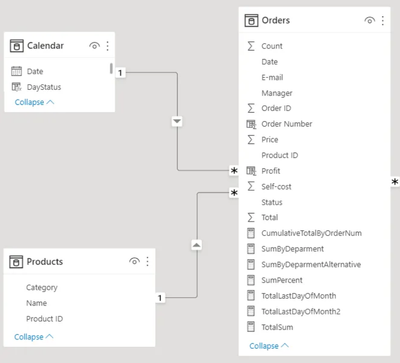To do this, let's create a table via SUMMARIZEIn the first parameter, we specify the Orders table, which is where the ProductID column we need to aggregate is located. Next, we must specify the column by which we want to perform our aggregation. Let's group the table based on a column that will not be contained in the Orders table. We will use another table that has a relationship with the Orders table - the Products table. In the Products table, we have a [Category] column.

Let's summarize our orders by product category. To do this, in the second parameter we need to specify a column for grouping from another table.

In the SUMMARIZE function, to specify a column linked to a table, you don't need to specify any special linking functions, because the SUMMARIZE function is originally configured to be easier to work with. All the internal functions for linking are already built into the SUMMARIZE function.

As expected, our output is a table with a single column. To add a new calculated aggregation column, where the information will be summarized by sales for each product, let's specify the name of our column ProductTotal and then the code of the created column SUM within which we specify the Total column from the Orders table. As a result, the corresponding column will be created in this summary table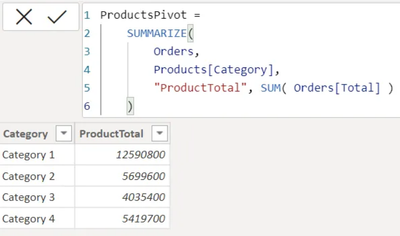Now in this summary table we need to aggregate information not only by product category, but also by product name, i.e. group our sales by both category and product name. To do this, we need to add another column for grouping before the column with aggregation.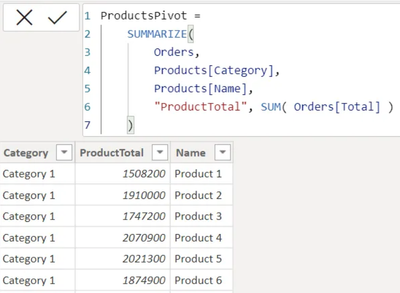Our column was added after all the columns - this is the DAX property. This is because we first created a Category column, added a second ProductTotal column to it, and then added a third Product[Name] column to this table. And DAX has these columns in the order in which they were created. Even if we remove the Category column and then restore it, the order will still be the same. To change the order, copy this code and delete this table. Let's create it again, then the order will be correct.We have a summary table, but it has totals and subtotals. The Category and Name columns are needed to perform aggregation, now we need totals by product category.

The SUMMARIZE function can do this with additional keywords. To summarize the Category column, we need to additionally aggregate information on those columns that are in the data model, specifically the Category column. To summarize Category4 for the three products in this category, we need to wrap the product name column in a special function ROLLUPGROUP.Four more rows have been added, but they are at the end of the table. To make it more convenient, let's sort this table by the Category column. Let's customize sorting by ascending order.If we wrap another column in the ROLLUPGROUP function, we will get duplicate rows by product with such initial dataIf the ROLLUPGROUP function wraps not only the Name column, but also the Category column, it will add another row with the total.If we assume that we don't need subtotals in the source table, but only the total, we can turn off subtotals by wrapping ROLLUPGROUP in the ROLLUP function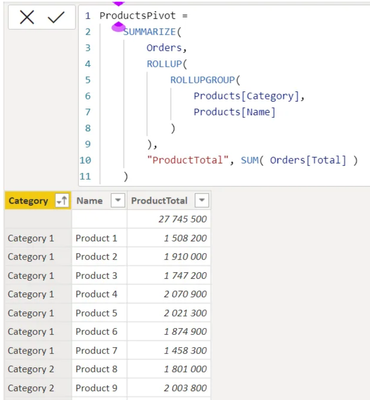Thus we get the total without summarizing.

Let's look at an example of using totals without summarizing table data.

As an option - it is necessary to address them from the DAX code. You can create such a table in DAX code and then refer directly to these values. In DAX you can specify the value of a particular cell, if you filter all rows and remove all unnecessary columns. This leaves one row with one cell, and one cell is one value.

To filter the table, you need to use the FILTER function. In the FILTER function you should specify that you want to return only those rows in which the Category column and Name column gives an empty value, then the FILTER function will return a table of one row.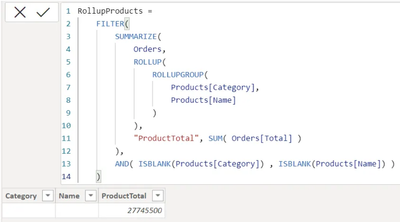Then we can optionally convert this table containing three columns into a table containing one column.

As we understand multiple columns cannot be converted to a scalar value. If we return not multiple columns, it turns out that DAX can convert one column and one row into one scalar value. Let's fix this error.

Let's use the SUMMARIZE functions, which by its indirect properties can return a single column. Let's wrap the filtered table into the SUMMARIZE function again. In the first parameter of the function we will specify the whole filtered table, and in the second parameter we will specify the column by which we want to group all the information - it is the ProductTotal column.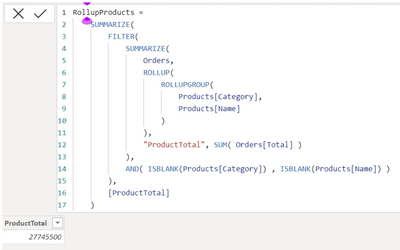Based on this code, a table with one row and one column was returned. The top function SUMMARIZE outputs a unique value from the created ProductTotal column based on our filtered table data.

Among other things, we can use this value to calculate the measure and output the visualization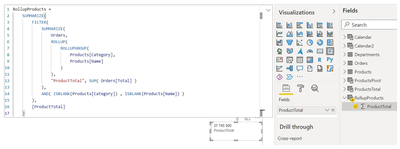Top Kudoed Posts
Latest Articles
Archives
Polls
What is your favorite Power BI feature release for September 2023?